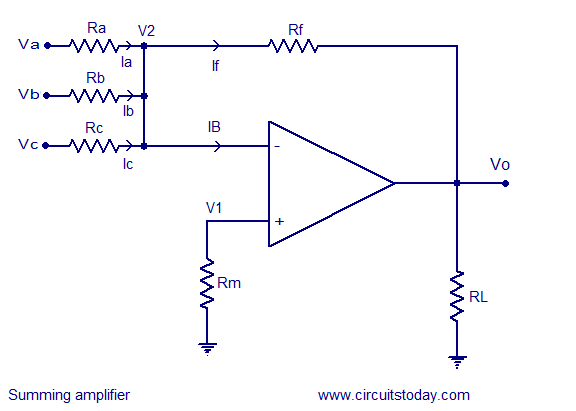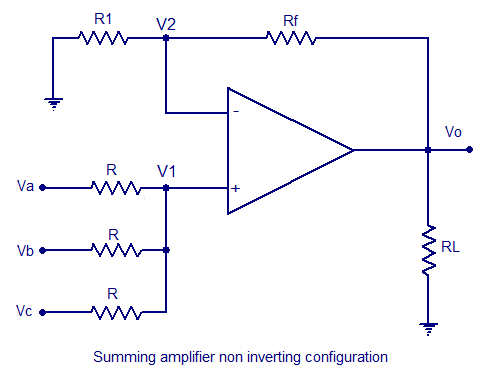## Audio

[Audio][slideshow]

## Summing amplifier using opamp.

Summing amplifier is a type operational amplifier circuit which can be used to sum signals. The sum of the input signal is amplified by a certain factor and made available at the output .Any number of input signal can be summed using an opamp. The circuit shown below is a three input summing amplifier in the inverting mode.Summing amplifier circuit

In the circuit, the input signals Va,Vb,Vc are applied to the inverting input of the opamp through input resistors Ra,Rb,Rc. Any number of input signals can be applied to the inverting input in the above manner. Rf is the feedback resistor.Non inverting input of the opamp is grounded using resistor Rm. RL is the load resistor. By applying kirchhoff’s current law at not V2 we get,

Ia+Ib+Ic = If+Ib

Since the input resistance of an ideal opamp is close to infinity and has infinite gain. We can neglect Ib & V2
There for Ia+Ib+Ic = If ……….(1)

Equation (1) can be rewritten as

(Va/Ra) + (Vb/Rb)+ (Vc/Rc) = (V2-Vo)/Rf

Neglecting Vo,

we get Va/Ra + Vb/Rb + Vc/Rc = -Vo/Rf

Vo = -Rf ((Va/Ra)+(Vb/Rb)+(Vc/Rc))

Vo = -((Rf/Ra )Va + (Rf/Rb) Vb + (Rf/Rc) Vc)……..(2)

If resistor Ra, Rb, Rc has same value ie; Ra=Rb=Rc=R, then equation (2) can be written as

Vo = -(Rf/R) x (Va + Vb +Vc)…………….(3)

If the values of Rf and R are made equal , then the equation becomes,

Vo = -(Va + Vb +Vc)

Averaging Circuit : An averaging circuit can be made from the above circuit by making the all input resistor equal in value ie; Ra = Rb = Rc =R and the gain must be selected such that if there are m inputs, then Rf/R must be equal to 1/m.

Scaling amplifier : In a scaling amplifier each input will be multiplied by a different factor and then summed together. Scaling amplifier is also called a weighted amplifier. Here different values are chosen for Ra, Rb and Rc. The governing equation is Vo = -((Rf/Ra )Va + (Rf/Rb) Vb + (Rf/Rc) Vc).

Summing amplifier in non inverting configuration.Summing amplifier in non inverting configuration

A non inverting summing amplifier circuit with three inputs are shown above. The voltage inputs Va, Vb and Vc are applied to non inverting input of the opamp. Rf is the feedback resistor. The output voltage of the circuit is governed by the equation;

Vo = (1+ (Rf/R1)) (( Va+Vb+Vc)/3)

[Lights][grids]